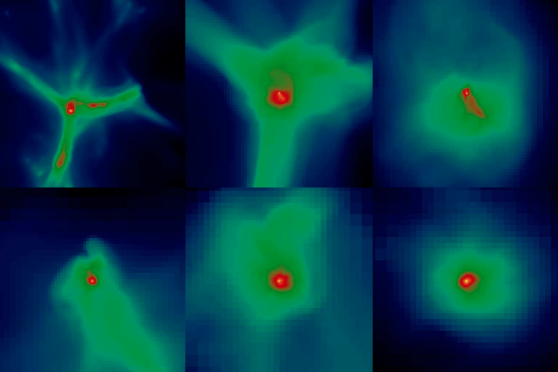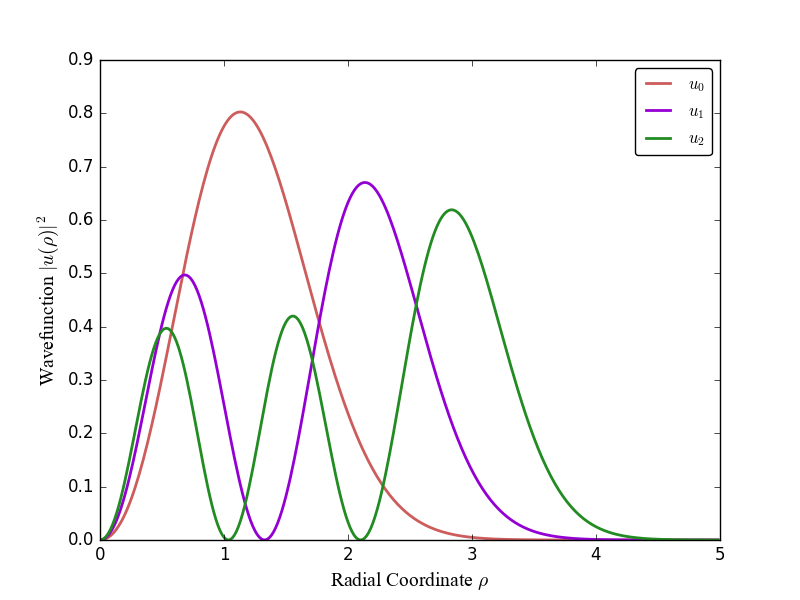### May 2017

The Chemistry of Star Formation### April 2016

Quantum Dots & Their Applications### December 2016

Schrödinger’s Equation for Two Electrons in a 3D Harmonic Oscillator Well

## Star Formation

While the formation of stars in the current universe is a well-researched phenomenon, little is known about how stars in the early universe might have formed. Since observation is not yet possible, advanced computer simulations (such as Enzo) must be used to model the primordial universe. These models can then be used to simulate Pop III star formation.

## Quantum Dots

A Quantum Dot is a semiconducting nanocrystal which tightly confines electrons in all spacial dimensions. These are a cutting-edge nanotechnology with anticipated applications in solar energy, quantum computing, light displays, nanomedicine, and more.

## Harmonic Oscillator

$-\dfrac{\hbar^2}{2m} \left( \dfrac{1}{r^2} \dfrac{d}{dr}r^2\dfrac{d}{dr} - \dfrac{\ell (\ell -1)}{r^2} \right) R(r) + V(r) R(r) = E R(r) \;\; , \;\; V(r) = \dfrac{m\omega^2 r^2}{2}$ The Schrödinger equation for one confined electron is a relatively simple example of a particle in a potential well. The two-electron case however, becomes much more challenging due to the repulsive interaction between the electrons. The linear algebra can become complicated to program, so using advanced pre-written libraries like Armadillo or BLAS is useful.